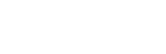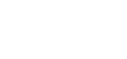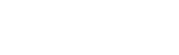# MATHEMATICS - Form 1 End of Term 1 2019 Examinations

SECTION 1: ANSWER ALL THE QUESTIONS (50MARKS)

1. Without using tables or calculator evaluate   (3mks)1. Three cups can hold 400ml, 450ml and 560ml of milk respectively. Find the capacity of the largest glass that can be used to fill each of the cups in full measure.
(3 marks)
1. A number n is such that when it is divided by 27, 30 or 45 the remainder is 3.
Find the smallest possible value of n.   (4mks)
1. Find the greatest number which when divided by 181 and 236 leave a remainder of five in each case.   (3mks
1. The G,C.D of two numbers is 7 and their L.C.M is 140. If one of the number is 20, find the other number.   (3mks)
1. (a) List down all prime number between 1 and 10 in descending order to form a number. (2mks)

(b) Find the total value of digit 5 in the number formed in (a) above   (2mks)

1. Evaluate (3mks)1. Evaluate without using tables or calculator.  (4mks)1. Write the following fraction in descending order  (2mks)2. Which of the following numbers are divisible by the numbers in bracket, give a reason in each case.
1. 270, 430, 510   (3)                          (2mks)
Reason _______________
2. 9904, 44344, 348544 (8)                 (2mks)
Reason _______________
1. Express the recurring decimals below as fraction   (4mks)
. .
0.32
1. Write the following numbers in words.   (2mks)
1. 543,654,444
2. 75, 356, 765, 003
1. Write the following numbers in figures. Nine hundred billion, two hundred and one million, ninety thousand, four hundred and ten.  (3mks)
1. To the nearest number indicated in brackets, round off each of the following numbers.      (3mks)
1. 607, 890, 567 (1 000)
2. 65, 745, 344 (10 000)
3. 146, 789 (100 000)
1. Express  5/7+ 7/12  as a decimal. Correct your answer to 3 decimal places. (3mks)
1. Write down the value of      (3mks)
1. (-3) x (-5)
2. (+8) x (-5)
3. (-40) ÷ (+8)

SECTION 2: ANSWER ALL THE QUESTIONS (5O MARKS)

1. Work out

6789 ÷ 13        (3mks)
2. From the above calculation, determine the
1. Quotient      (1mk)
2.  Remainder      (1mk)
3. Prove that the divided is 6789 in the work out above.   (3mks)
4. Convert the following into improper fraction. 34/   (2mks)
1.
1. Express the following numbers as product of prime numbers. (leave your answer in power form) (6mks)
1. 100
2. 1080
3. 588
Find
1. The L.C.M of the numbers leaving your answer in power form. (2mks)
2. The G.C.D of the numbers leaving your answer in power form. (2mks)
1.
1. Three friends, Betty, Ann tabby and Tracy decide to buy a car. Betty pays a quarter of the cost, Ann tabby pays a third of the cost and Tracy pays the rest. If Tracy pays sh150000. Calculate;
1. The cost of the car.    (4mks)
2. How much Betty pays (2mks)
2. Brian uses 2/3 of his land grazing, 1/of the remainder for planting potatoes and 1/10 of what is left for other farming activities. If 6.3 hectares of land is unused, determine the size of the land he owns.    (4mks)
2.
1. A minibus had 25 passengers at the beginning of a journey. Fourteen passengers alighted at the first stop while 11 boarded. Eight of those who boarded at the first stop alighted at the second stop and 14 got in. The minibus did not stop again up to the final destination. The charges from the starting point were sh60 p to first stop, sh80 up to the second stop and sh95 up to the final destination.
1. How many passengers alighted at the final destination?  (2mks
2. How many passengers were ferried by the minibus through the journey? (2mks)
3. How much money was collected during the trip?    (4mks)
2. A television dealer sold TV set for a total of sh.388 500 at sh18 500 per set.

How many TV set were sold?  (2mks)

1. Perform the following operations using a number line and state the answer.
1. (-4) + (-2) (2mks)
2. (-7) - (-8)  (2mks)
3. (+5) -(-2)   (2mks)
4. (+1) + (+4)   (2mks)
5. (+5) - (+7)   (2mks)

#### Download MATHEMATICS - Form 1 End of Term 1 2019 Examinations.

• ✔ To read offline at any time.
• ✔ To Print at your convenience
• ✔ Share Easily with Friends / Students